Online Tution   »   CBSE Syllabus - Class 9,10, 11,...   »   CBSE Class 9 Maths Syllabus 2022-...

# CBSE Class 9 Maths Syllabus 2022- Check NCERT Syllabus & PDF

## Class 9 Syllabus 2022-23 Maths

Maths is a subject which requires practice and to start preparation for CBSE Class 9 Maths the students must have CBSE Class 9 Mathematics Syllabus 2023 beforehand. First, the students must complete all the units given in CBSE Class 9 Mathematics Syllabus 2023. After completing the CBSE Class 9 Mathematics Syllabus 2023 the students must practice all the examples and practice questions given in the book. To get good marks in Class 9 Maths the students can solve the practice paper and the previous year’s question paper after completing CBSE Class 9 Mathematics Syllabus 2023. Bookmark this page to get all the latest updates from the Central Board of Secondary Education.

## Maths Class 9 Syllabus 2022-23- Unit wise

Below we have given the unit wise mark distribution in CBSE Class 9 Mathematics Syllabus 2023. CBSE Class 9 Mathematics Syllabus 2023 covers Number systems, Algebra, Coordinate Geometry, Geometry, Mensuration, Statistics and Probability. Check the other information in the table below:

 Unit Name Marks Unit 1: Number System 10 Unit 2: Algebra 20 Unit 3: Coordinate Geometry 04 Unit 4: Geometry 27 Unit 5: Mensuration 13 Unit 6: Statistics and Probability 06 Total 80

## CBSE Class 9 Syllabus Maths 2022-23: Full NCERT Syllabus Unit 1

Unit 1: Number Systems

Real Numbers
1. Review of representation of natural numbers, integers, and rational numbers on the number line. Rational numbers as recurring/ terminating decimals. Operations on real numbers.
2. Examples of non-recurring/non-terminating decimals. Existence of non-rational numbers (irrational numbers) such as √2, √3 and their representation on the number line. Explaining that every real number is represented by a unique point on the number line and conversely, viz. every point on the number line represents a unique real number.
3. Definition of nth root of a real number.
4. Rationalization (with precise meaning) of real numbers of the type 1/a+b√x  and 1/√x+√y (and their combinations) where x and y are natural numbers and a and b are integers.
5. Recall of laws of exponents with integral powers. Rational exponents with positive real bases (to be done by particular cases, allowing the learner to arrive at the general laws.)

## CBSE Class 9 Syllabus Maths 2022-23: Full NCERT Syllabus Unit 2

Unit 2: Algebra

1. Polynomials
Definition of a polynomial in one variable, with examples and counter examples. Coefficients of a polynomial, terms of a polynomial and zero polynomial. Degree of a polynomial. Constant, linear, quadratic and cubic polynomials. Monomials, binomials, trinomials. Factors and multiples. Zeros of a polynomial. Motivate and State the Remainder Theorem with examples. Statement and proof of the Factor Theorem. Factorization of ax2+ bx + c, a ≠ 0 where a, b and c are real numbers, and of cubic polynomials using the Factor Theorem. Recall of algebraic expressions and identities. Verification of identities: and their use in factorization of polynomials.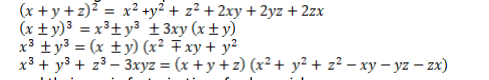2. Linear Equations in Two Variables

Recall of linear equations in one variable. Introduction to the equation in two variables. Focus on linear equations of the type ax + by + c=0.Explain that a linear equation in two variables has nfinitely many solutions and justify their being written as ordered pairs of real numbers, plotting them and showing that they lie on a line.

## CBSE Class 9 Syllabus Maths 2022-23: Full NCERT Syllabus Unit 3

Unit 3: Coordinate Geometry

The Cartesian plane, coordinates of a point, names and terms associated with the coordinate plane, notations.

## CBSE Class 9 Syllabus Maths 2022-23: Full NCERT Syllabus Unit 4

Unit 4: Geometry

1. Introduction to Euclid’s Geometry

History – Geometry in India and Euclid’s geometry. Euclid’s method of formalizing observed phenomenon into rigorous Mathematics with definitions, common/obvious notions, axioms/postulates and theorems. The five postulates of Euclid. Showing the relationship between axiom and theorem, for example:
(Axiom) 1. Given two distinct points, there exists one and only one line through them.
(Theorem) 2. (Prove) Two distinct lines cannot have more than one point in common.

2. Lines and Angles

1. (Motivate) If a ray stands on a line, then the sum of the two adjacent angles so formed is 180 degrees and the converse.
2. (Prove) If two lines intersect, vertically opposite angles are equal.

3. (Motivate) Lines which are parallel to a given line are parallel.

3. Triangles

1. (Motivate) Two triangles are congruent if any two sides and the included angle of one triangle is equal to any two sides and the included angle of the other triangle (SAS Congruence).

2. (Prove) Two triangles are congruent if any two angles and the included side of one triangle is equal to any two angles and the included side of the other triangle (ASA Congruence).

3. (Motivate) Two triangles are congruent if the three sides of one triangle are equal to three sides of the other triangle (SSS Congruence).

4. (Motivate) Two right triangles are congruent if the hypotenuse and a side of one triangle are equal (respectively) to the hypotenuse and a side of the other triangle. (RHS Congruence)

5. (Prove) The angles opposite to equal sides of a triangle are equal.

6. (Motivate) The sides opposite to equal angles of a triangle are equal.

1. (Prove) The diagonal divides a parallelogram into two congruent triangles.

2. (Motivate) In a parallelogram opposite sides are equal, and conversely.

3. (Motivate) In a parallelogram opposite angles are equal, and conversely.

4. (Motivate) A quadrilateral is a parallelogram if a pair of its opposite sides is parallel and equal.

5. (Motivate) In a parallelogram, the diagonals bisect each other and conversely.

6. (Motivate) In a triangle, the line segment joining the mid points of any two sides is parallel to the third side and in half of it and (motivate) its converse.

5. Circles

1.(Prove) Equal chords of a circle subtend equal angles at the center and (motivate) its converse.

2.(Motivate) The perpendicular from the center of a circle to a chord bisects the chord and conversely, the line drawn through the center of a circle to bisect a chord is perpendicular to the chord.

3. (Motivate) Equal chords of a circle (or of congruent circles) are equidistant from the center (or their respective centers) and conversely.

4.(Prove) The angle subtended by an arc at the center is double the angle subtended by it at any point on the remaining part of the circle.

5.(Motivate) Angles in the same segment of a circle are equal.

6.(Motivate) If a line segment joining two points subtends equal angle at two other points lying on the same side of the line containing the segment, the four points lie on a circle.

7.(Motivate) The sum of either of the pair of the opposite angles of a cyclic quadrilateral is 180° and its converse.

## CBSE Class 9 Syllabus Maths 2022-23: Full NCERT Syllabus Unit 5

Unit 5: Mensuration

1. Areas: Area of a triangle using Heron’s formula (without proof)

2. Surface Areas and Volumes: Surface areas and volumes of spheres (including hemispheres) and right circular cones.

## CBSE Class 9 Syllabus Maths 2022-23: Full NCERT Syllabus Unit 6

Unit 6: Statistics and Probability

Statistics: Bar graphs, histograms (with varying base lengths), and frequency polygons.

## CBSE Class 9 Syllabus Maths 2022-23: NCERT Question Paper Design

Here we have given the question paper design of CBSE Class 9 Mathematics. In the below table, we have given the marks distribution and weightage given in CBSE Class 9 Mathematics Syllabus 2023. Check out the table given below:

 S. No. Typology of Questions Total Marks Weightage(%) 1. Remembering: Exhibit memory of previously learned material by recalling facts, terms, basic concepts, and answers. Understanding: Demonstrate understanding of facts and ideas by organizing, comparing, translating, interpreting, giving descriptions, and stating main ideas 43 54 2. Applying: Solve problems to new situations by applying acquired knowledge, facts, techniques and rules in a different way. 19 24 3. Analysing: Examine and break information into parts by identifying motives or causes. Make inferences and find evidence to support generalizations Evaluating: Present and defend opinions by making judgments about information, validity of ideas, or quality of work based on a set of criteria. Creating: Compile information together in a different way by combining elements in a new pattern or proposing alternative solutions 18 22 Total 80 100

## CBSE Class 9 Maths Syllabus 2023: Internal Assessment

The internal assessment of CBSE Class 9 maths is given in the table below. As per the CBSE Class 9 Mathematics Syllabus 2022, the internal assessment will be based on Pen Paper Test and Multiple Assessment, Portfolio, and Lab Practical (Lab activities to be done from the prescribed books). Check the marks distribution given in the table below:

 Internal Assessment Marks Pen Paper Test and Multiple Assessment (5+5) 10 Portfolio 5 Lab Practical (Lab activities to be done from the prescribed books) 5 Total 20

## NCERT Books for CBSE Class 9 Maths Syllabus 2022-23

Below we have given the reference books for CBSE Class 9 Mathematics. The students must check out the books given below and start their preparation:

1. Mathematics – Textbook for Class IX – NCERT Publication
2. Guidelines for Mathematics Laboratory in Schools, class IX – CBSE Publication
3. Mathematics exemplar problems for class IX, NCERT publication.

## CBSE Class 9 Maths Syllabus 2022-23: FAQs

Q. Will CBSE conduct CBSE Class 9 Exam 2023 annually?
Yes, CBSE has recently announced that from the next session CBSE will conduct annual board exams.
Q. Where I can get CBSE Class 9 Mathematics Syllabus 2023?
You can get CBSE Class 9 Mathematics Syllabus 2023 from this page. To get the syllabus of other subjects the students can visit the official website of Adda247 School.
Q. How many units are there in CBSE Class 9 Mathematics Syllabus 2023?
There are 6 units in CBSE Class 9 Mathematics Syllabus 2023. The CBSE Class 9 Mathematics Syllabus 2023 covers Number systems, Algebra, Coordinate Geometry, Geometry, Mensuration, Statistics and Probability.

Sharing is caring!

## FAQs

### Will CBSE conduct CBSE Class 9 Exam 2023 annually?

Yes, CBSE has recently announced that from the next session CBSE will conduct annual board exams.

### Where I can get CBSE Class 9 Mathematics Syllabus 2023?

You can get CBSE Class 9 Mathematics Syllabus 2023 from this page. To get the syllabus of other subjects the students can visit the official website of Adda247 School.

### How many units are there in CBSE Class 9 Mathematics Syllabus 2023?

There are 6 units in CBSE Class 9 Mathematics Syllabus 2023. The CBSE Class 9 Mathematics Syllabus 2023 covers Number systems, Algebra, Coordinate Geometry, Geometry, Mensuration, Statistics and Probability.

### TOPICS:

•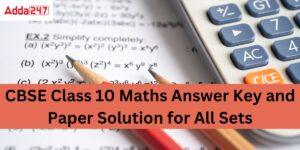Class 10 Maths Answer Key 2023, Set 1,2,...
•CBSE Class 12 Informatics Practices Syll...
•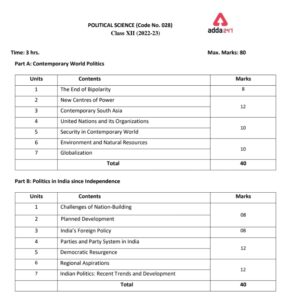Political Science Class 12 Syllabus 2022...
•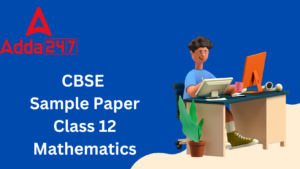Maths Sample Paper Class 12 2023 with CB...
•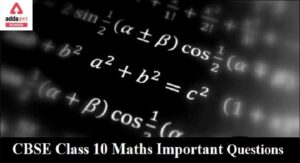CBSE Important Questions for Class 10 Ma...
•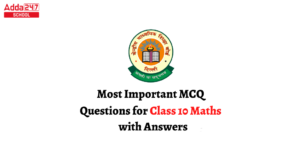CBSE Class 10 Maths MCQ Questions With A...